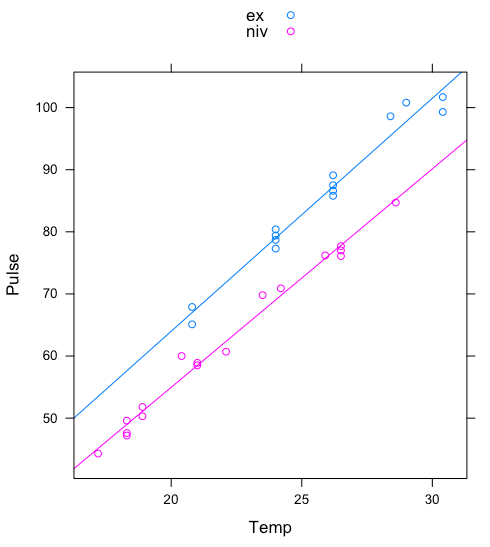# Analysis of Covariance

Often we are dealing with experiments or studies in which more than one treatment is applied simultaneously and we want to know what’s their relative effect on the response variable. In case we are manipulating a continuous variable and a categorical one, the parametric analysis traditionally used to assess their relative importance is the Analysis of Covariance (ANCOVA).

Example: let’s consider the chirps of two cricket species and check if their pulse rate differ across a temperature gradient. This is a study that was actually performed by Walker (1962) on species Oecanthus exclamationis and Oecanthus niveus.Graph displaying the response of pulse at different temperatures (continuous explanatory variable) in two different species (species identity is the categorical variable).
``````# data from: https://rcompanion.org/rcompanion/e_04.html
Input = ("
Species Temp Pulse
ex 20.8 67.9
ex 20.8 65.1
ex 24 77.3
ex 24 78.7
ex 24 79.4
ex 24 80.4
ex 26.2 85.8
ex 26.2 86.6
ex 26.2 87.5
ex 26.2 89.1
ex 28.4 98.6
ex 29 100.8
ex 30.4 99.3
ex 30.4 101.7
niv 17.2 44.3
niv 18.3 47.2
niv 18.3 47.6
niv 18.3 49.6
niv 18.9 50.3
niv 18.9 51.8
niv 20.4 60
niv 21 58.5
niv 21 58.9
niv 22.1 60.7
niv 23.5 69.8
niv 24.2 70.9
niv 25.9 76.2
niv 26.5 76.1
niv 26.5 77
niv 26.5 77.7
niv 28.6 84.7")

str(dd)
names(dd) <- c("Species","Temp","Pulse") # make column names more readable``````

Let’s display the data graphically in a quick and dirty way:

``````library(lattice)
xyplot(Pulse ~ Temp, groups=Species, data=dd, type=c("p", "r"), auto.key=T)``````I would not use this figure in a publication but it’s perfectly good for exploring the data.
``````# Model selection:

lm1 <- lm(Pulse ~ Temp*Species, data=dd) # interactive model
lm2 <- lm(Pulse ~ Temp+Species, data=dd) # additive model
lm3 <- lm(Pulse ~ Temp, data=dd) # temperature matters, species id. does not
lm4 <- lm(Pulse ~ Species, data=dd) # species id. matters, temperature does not
lm0 <- lm(Pulse ~ 1, data=dd) # null model:
# neither temperature nos sp. id. matter in predicting pulse

# alternatively compare models with an F test:
anova(lm1, lm2) # if there's no significant difference retain the simplest model, in this case lm2
anova(lm2, lm3) # lm2 explains a significantly larger amount of the variability observed in the data compared to lm3. Notice that RSS, which is a proxy of the residual variability left unaccounted for by the model, is smaller in lm2.
summary(lm1)
summary(lm2)``````

An alternative tool for model selection is the Akaike Information Criterion (AIC). Here are some considerations on AIC by Brian McGill. The AIC estimates the goodness of a model by measuring how well the model fit the data, while penalising models according to their number of parameters. In other words, it favours the simplest model that describes the data best. The lower the AIC, the better the model. For small sample sizes the corrected AIC (AICc) should be used. See: `AICc()` in package `MuMIn`. For large sample sizes, AICc ~ AIC. The minimum AIC difference for a model to be considered significantly better at describing the data is debated; Harrison et al. (2018) suggest ∆AIC=6. For competing models with ∆AIC<6, I consider the simplest to be the best.

Let’s plot a nicer graph with plot():

``````# first subset by species:
unique(dd\$Species)
ex <- subset(dd, dd\$Species=="ex")
niv <- dd[dd\$Species=="niv",]
# this normally work... I think the species names have some spaces before or after the visible characters.
# this way will work:
ex <- subset(dd, dd\$Species==unique(dd\$Species))
niv <- dd[dd\$Species==unique(dd\$Species),]
# run a regression for each:
lm_ex <- lm(Pulse~Temp, data=ex)
lm_niv <- lm(Pulse~Temp, data=niv)
# plot the first subset
plot(Pulse~Temp, data=ex,
xlim=c(16,33), ylim=c(40,105),
pch=19, # or 16
col="red"
)
points(Pulse~Temp, data=niv)
# plot regression lines:
abline(lm_ex,
lty="solid", col="red"
)
abline(lm_niv,
lty="dashed", col="black"
)
legend("topleft", title="Species:",
legend=unique(dd\$Species),
pch=c(19,1),
lty=c("solid", "dashed"),
col=c("red", "black")
)``````

Re-draw the figure making sure that the regression lines only predict values within the range of observed values:

``````# Set a sequence of hypothetic values for the explanatory variable:
temps_ex <- data.frame(Temp=seq(min(ex\$Temp), max(ex\$Temp), 0.01))
# This just helps keeping the following lines of code short and clean:
texT<- temps_ex\$Temp
# predict values with standard error
yy_ex<- predict(lm_ex, data.frame(x=temps_ex), type="response", se=T)

# do the same for niv:
temps_niv <- data.frame(Temp=seq(min(niv\$Temp), max(niv\$Temp), 0.01))
tnivT<- temps_niv\$Temp
yy_niv<- predict(lm_niv, data.frame(x=temps_niv), type="response", se=T)

plot(Pulse~Temp, data=ex,
xlim=c(16,33), ylim=c(40,105),
xlab="Temperature",
pch=NA
) # this plots a blank graph
# plot points:
points(Pulse~Temp, data=ex,
pch=19, # or 16
col="red"
)
points(Pulse~Temp, data=niv)
# plot fitted models:
# I'm using points() again but with the argument type="l", which plots lines
points(x=texT,y=yy_ex\$fit, type="l",
lty="solid", col="red"
)
points(x=tnivT,y=yy_niv\$fit, type="l",
lty="dashed", col="black"
)
legend("topleft", title="Species:",
legend=unique(dd\$Species),
pch=c(19,1),
lty=c("solid", "dashed"),
col=c("red", "black")
)``````

``````# this just helps keeping the following lines of code short and clean:
yysep_ex <- yy_ex\$fit+1.96 * yy_ex\$se
yysem_ex <- yy_ex\$fit-1.96 * yy_ex\$se
# Again, this just helps keeping the following lines of code short and clean:
yysep_niv <- yy_niv\$fit+1.96 * yy_niv\$se
yysem_niv <- yy_niv\$fit-1.96 * yy_niv\$se

# Confidence interval as a shaded area:

polygon(c(texT,rev(texT)),c(yysep_ex,rev(yysem_ex)), col= scales::alpha("red", 0.2), border=NA)
polygon(c(tnivT,rev(tnivT)),c(yysep_niv,rev(yysem_niv)),col=scales::alpha("gray", 0.4), border=NA)``````

For transparent colors use scales::alpha, namely function alpha() in package scales.

• Here I show how to interpret the results of a slightly more complicated example, with three explanatory variables: two categorical and one continuous.
• multcomp is an R package that can perform post-hoc pairwise comparisons for ANCOVA designs.